# TARGET SBI EXAM 2018: DATA INTERPRETATION

Dear Bankersdaily Aspirants,

Hope , Your preparation for SBI Clerk & PO exam 2018 is good . Since SBI Clerk & PO exam is a Two level which will be conducted in an online mode, Competition will be higher for this SBI Clerk & PO Exam and the most important thing , Current affairs section will be there in this exam. So aspirants have to concentrate in Four sections namely Aptitude, Reasoning, English, Current Affairs.

The person with high speed and very good accuracy only can crack this exam to taste the success. The candidates will be selected on the basis of performance in this online exam test. So the candidate with consistent hard work and regular practice will crack the exam very easily.

Exam: SBI  Clerk & PO Exam 2018

Topic: Data Interpretation

Day: 29

Timing: 7 minutes

D.1 – 5 ) Study the following information carefully and answer the given questions

The following chart shows the breakup of students in five different colleges

Total number of students in college C is 4200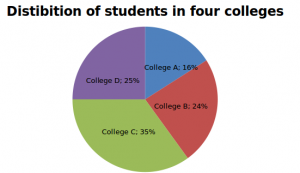Male to female ratio Male Female College A 11 13 College B 11 5 College C 2 5 College D 1 1

Q.1) What is the total number of male students in colleges A and B together?

a) 2680

b) 2860

C) 2660

d) 3680

e) 2600

Q.2) What is the difference between the total number of female students in college D and the total number of male students in college C?

a) 301

b) 310

c) 200

d) 300

e) 320

Q.3) What is the ratio between the total number of female students in College C and D together to the total number of students in colleges A and B together?

a) 11:12

b) 12:13

c) 16:15

d) 14:15

e) 15:16

Q.4) Total number of male students in all the colleges is what percent of the total number of students in College C and D together?

a) 74.23%

b) 78.99%

c) 77.22%

d) 89%

e) 87.22%

Q.5) In College E, total number of students equals to 95% of the total number of students in college D. Male to female ratio is 1:2. Then find the number of female students in College E

a) 2000

b) 1700

c) 1900

d) 1950

e) 2850

### D.(6 – 10 ) Study the following table carefully to answer that follow.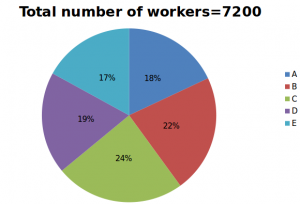M:F denotes ratio of male to female workers in each department

 (DEPARTMENT M:F A 5:3 B 5:7 C 3:5 D 11:8 E 9:8

Q.6) what is the total number of male workers and female workers working in department C and E respectively?

a) 684

b) 948

c) 1224

d) 1430

e) None of these

Q.7) what is the ratio of male workers in department A to female workers in department B?

a)153:154

b) 135:143

c) 135:137

d) 135:154

e) None of these

Q.8) Male workers of department A is what percent of the female workers in department C?

a) 55

b) 75

c) 65

d) 83

e) None of these

Q.9) Male workers of department B is what percent more than that of female workers in department D?

a) 14.58%

b) 18.45%

c) 25%

d) 32.25%

e) None of these

Q.10) What is the sum of average of male workers and average of female workers in all the departments?

a) 735

b) 811.6

c) 1321.5

d) 1440

e) None of these

1) b) 2860

 Total number of students Total number of male students Total number of female students College A 1920 880 1040 College B 2880 1980 900 College C 4200 1200 3000 College D 3000 1500 1500

Total male students in colleges A and B=880+1980=2860

2) d) 300

 Total number of students Total number of male students Total number of female students College A 1920 880 1040 College B 2880 1980 900 College C 4200 1200 3000 College D 3000 1500 1500

Difference=1500-1200=300

3) e) 15:16

 Total number of students Total number of male students Total number of female students College A 1920 880 1040 College B 2880 1980 900 College C 4200 1200 3000 College D 3000 1500 1500

Ratio=(3000+1500):(1920+2880)=4500:4800=45:48=15:16

4) c) 77.22%

 Total number of students Total number of male students Total number of female students College A 1920 880 1040 College B 2880 1980 900 College C 4200 1200 3000 College D 3000 1500 1500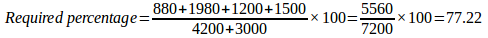5) c) 1900

In college E total students=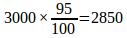Female students=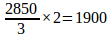6) c) 1224

Total Number of workers in each department

A = 1296

B = 1584

C = 1728

D = 1368

E = 1224

 DEPARTMENT M:F A 810:486 B 660:924 C 648:1080 D 792:576 E 648:576

Total number of male workers in department C = 648

Total number of female workers in department E = 576

Total = 648 + 576 = 1224

7) d) 135:154

Total Number of workers in each department

A = 1296

B = 1584

C = 1728

D = 1368

E = 1224

 DEPARTMENT M:F A 810:486 B 660:924 C 648:1080 D 792:576 E 648:576

Male workers in department A = 810

Female workers in department B = 924

810 : 924 = 405 : 462 = 135 : 154

8) b) 75

Total Number of workers in each department

A = 1296

B = 1584

C = 1728

D = 1368

E = 1224

 DEPARTMENT M:F A 810:486 B 660:924 C 648:1080 D 792:576 E 648:576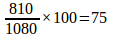9) a) 14.58%

Total Number of workers in each department

A = 1296

B = 1584

C = 1728

D = 1368

E = 1224

 DEPARTMENT M:F A 810:486 B 660:924 C 648:1080 D 792:576 E 648:576

Male workers of department B = 660

Female workers of department D = 576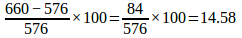10) d) 1440

Total Number of workers in each department

A = 1296

B = 1584

C = 1728

D = 1368

E = 1224

 DEPARTMENT M:F A 810:486 B 660:924 C 648:1080 D 792:576 E 648:576

Average of male workers = (810+660+648+792+648) = 3558/5= 711.6

Average of female workers = (486+924+1080+576+576) = 3642/5 = 728.4

Total Average = 711.6 + 728.4 = 1440

 Day 29 MOT Data Interpretation Sentence rearrangement (new)

Aspirants can also check the other topics from the SBI CLERK STUDY PLANNER from the link given below. DObookmark the given link as all the posts will be updated in that page and aspirants can check the daily updates from this page or from the Homepage of Bankersdaily.